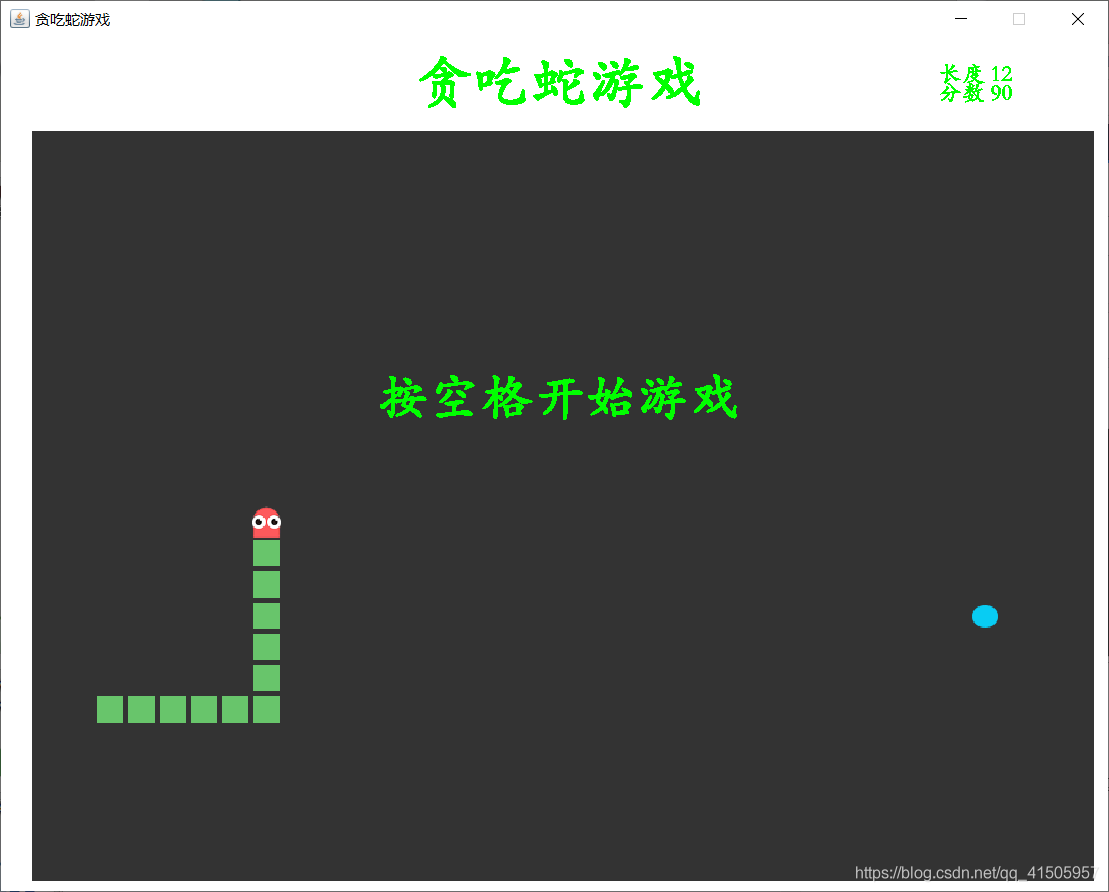﻿ java实现贪吃蛇游戏代码(附完整源码)_java_脚本之家
java# java实现贪吃蛇游戏代码(附完整源码)## GUI界面

java实现贪吃蛇游戏需要创建一个桌面窗口出来，此时就需要使用java中的swing控件

```JFrame frame = new JFrame("贪吃蛇游戏");
//设置大小
frame.setBounds(10, 10, 900, 720);```

```public static URL bodyUrl = GetImage.class.getResource("/picture/body.png");
public static ImageIcon body = new ImageIcon(bodyUrl);```

```//每次刷新页面需要进行的操作
@Override
public void actionPerformed(ActionEvent e) {
//当游戏处于开始状态且游戏没有失败时
if(gameStart && !isFail) {
//蛇头所在的位置就是下一次蛇身体的位置

//bodyIndexLeft++;
//长度到达数组的尾部
if(bodyIndexRight==480) {
for(int i=bodyIndexLeft, j=0; i<=bodyIndexRight; i++,j++) {
bodyX[j]=bodyX[i];
bodyY[j]=bodyY[i];
}
bodyIndexLeft=0;
bodyIndexRight=length-1;
}
//更新头部位置
if(fdirection==1) {
//头部方向为上，将蛇头向上移动一个单位
}
else if(fdirection==2) {
//头部方向为下，将蛇头向下移动一个单位
}
else if(fdirection==3) {
//头部方向为左，将蛇头向左移动一个单位
}
else if(fdirection==4) {
//头部方向为右，将蛇头向右移动一个单位
}

//当X坐标与Y坐标到达极限的时候，从另一端出来

//当头部坐标和食物坐标重合时
length++;
score+=10;
//重新生成食物,判断食物坐标和蛇身坐标是否重合，效率较慢
while(true) {
foodX = 25 + 25* random.nextInt(34);
foodY = 75 + 25* random.nextInt(24);

//判断食物是否和头部身体重合
boolean isRepeat = false;
//和头部重合
isRepeat = true;
//和身体重合
for(int i=bodyIndexLeft; i<=bodyIndexRight; i++) {
if(foodX == bodyX[i] && foodY == bodyY[i]){
isRepeat = true;
}
}

//当不重复的时候，食物生成成功，跳出循环
if(isRepeat==false)
break;
}

}
else bodyIndexLeft++;

//判断头部是否和身体重合
for(int i=bodyIndexLeft; i<=bodyIndexRight;i++) {
//游戏失败
isFail = true;
break;
}
}
repaint();
}

timer.start();
}```

## 键盘监听

```//键盘监听事件
@Override
public void keyPressed(KeyEvent e) {
int keyCode = e.getKeyCode(); //获取按下的键盘

if (keyCode==KeyEvent.VK_SPACE){ //如果是空格
if (isFail){ //如果游戏失败,从头再来！
isFail = false;
init(); //重新初始化
}else { //否则，如果游戏中，暂停游戏，如果暂停游戏，开始游戏
gameStart = !gameStart;
}
repaint();
}

if (keyCode==KeyEvent.VK_LEFT){
//左
//不能掉头,当当前头的方向和要转的方向不相反时才能调转方向
if(fdirection!=4) {
fdirection = 3;
}

}else if (keyCode==KeyEvent.VK_RIGHT){
//左
if(fdirection!=3)
fdirection = 4;
}else if (keyCode==KeyEvent.VK_UP){
//上
if(fdirection!=2)
fdirection = 1;
}else if (keyCode==KeyEvent.VK_DOWN){
//下
if(fdirection!=1)
fdirection = 2;
}
}```